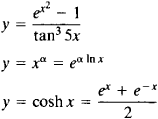# Elementary Function

Also found in: Wikipedia.

## elementary function

[‚el·ə′men·trē ′fəŋk·shən]
(mathematics)
Any function which can be formed from algebraic functions and the exponential, logarithmic, and trigonometric functions by a finite number of operations consisting of addition, subtraction, multiplication, division, and composition of functions.

## Elementary Function

any function in the class consisting of polynomials, rational functions, exponential functions, logarithmic functions, trigonometric functions, and inverse trigonometric functions. The class of elementary functions also includes functions obtained from those listed above through the performance of the four arithmetic operations and composition (the formation of a composite function) a finite number of times; examples areThe class of elementary functions has been best studied, and is most often encountered, in applications of mathematics. Many practical problems, however, lead to the consideration of functions that are not elementary functions, such as cylindrical functions. The derivative of an elementary function is also an elementary function. The indefinite integral of an elementary function is not always expressed in terms of an elementary function. Nonelementary functions are often represented in terms of elementary functions through the use of, for example, infinite series, infinite products, and infinite integrals.

References in periodicals archive ?
For each elementary function, we searched for at least one solution based on a literature review, analysis of similar systems in the market or patented systems, and application of the brainstorming method as proposed by BACK et al.
When a or b are positive integers, the incomplete beta function is an elementary function of z.
However, in several cases the coordinate transformation x(z) is inverted thus producing explicitly written potentials given as V = V(z(x)) through an elementary function z = z(x).
Now we shall call the function defined in (1) [mathematical expression not reproducible] since it does not refer to anyone and it has unknown analytic representation as elementary function using standard special functions and the RHS of (18) presents another representation of T(x) function using CDF of the normal distribution.
Therefore, one major consequence should be that the RTD function of this zone can theoretically be calculated by a proper but simple combination of the elementary function of the EZ or KN elements at the same feed rate and speed.
All educational components operate with mathematical functions that are implemented through one or more elementary Function objects.
Mr McKendrick said: "The elementary function of transferring money from one person to another and providing cash for wages and other purposes, in appropriate amounts, operates with easy efficiency, but banking is a much more intricate business than is indicated by the simple transactions passing over the banks' counters.
An elementary function is called basis and set of bases functions is a dictionary.
It is in general not an elementary function, unlike the related logarithm function.
We now turn our attention to describing the condition for the integrability for an elementary function. If f is an elementary function, f is differentiable at x, and we have
The Gabor coefficients [C.sub.m,n] represent the relative information content of the signal captured by the corresponding elementary function at each coordinate on the lattice.

Site: Follow: Share:
Open / Close